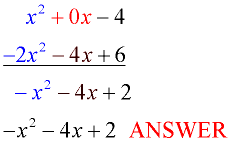Polynomials: Add & Subtract MathBitsNotebook.com Terms of Use   Contact Person: Donna Roberts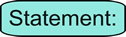To add or subtract polynomials, remove parentheses if any are present, and combine the like terms. Remember to be careful of the signs.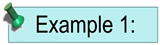Add: (3x2 + 5x - 3) + (x2 - 5x + 1)Horizontal Method:
 (3x2 + 5x - 3) + (x2 - 5x + 1) = 3x2 + 5x - 3 + x2 - 5x + 1 (remove parentheses, identify the like terms) = 3x2 + x2 + 5x - 5x - 3 + 1 (group the like terms together) = (3 + 1)x2 + (5 - 5)x - 3 + 1 (use the reverse distributive property - factor) = 4x2 + 0x - 2 (add, being careful of the signs) = 4x2 - 2 ANSWER ( it is not necessary to write the middle term "+ 0x" )Vertical Method:
 Some people prefer to use a vertical method when adding or subtracting polynomials. Line up the polynomials so that the like terms are one under the other. It may be necessary to insert a 0 place holder if a term is missing.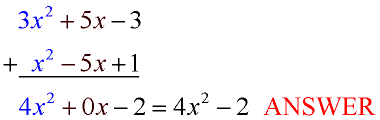Algebra Tiles: Algebra tiles give you a "visual" understanding of the process of polynomial addition or subtraction. Key: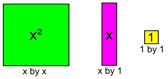Remember that red tiles represent negative values. See more about Algebra Tiles.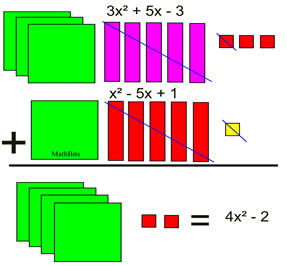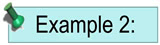Add: (3x + 4x2 - 9) + (-2x2 + 10)

 Horizontal Method: (3x + 4x2 - 9) + (-2x2 + 10) = 3x + 4x2 - 9 + -2x2 + 10 = 4x2 - 2x2 + 3x - 9 + 10 = 2x2 + 3x + 1 ANSWER Vertical Method: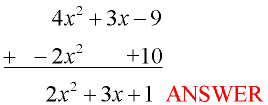This example shows placing the trinomial in standard form (powers listed in descending order) before adding. Notice how the terms were lined up under like terms.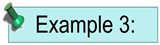Subtract (2x2 + 4x - 6) from (x2 - 4)

 Horizontal Method: Notice the order of this subtraction. Just as is done when subtracting signed numbers, change the signs of the terms being subtracted (or distribute -1), and follow the rules for adding signed like terms. (x2 - 4) - (2x2 + 4x - 6)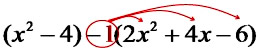(distribute -1) = x2 - 4 - 2x2 - 4x + 6 = x2 - 2x2 - 4x - 4 + 6 = -x2- 4x + 2 ANSWER Vertical Method: Again, notice the order of the subtraction. Change the signs of the terms being subtracted, and follow the rules for adding signed like terms. In this problem, we insert 0x to hold the missing column for x.